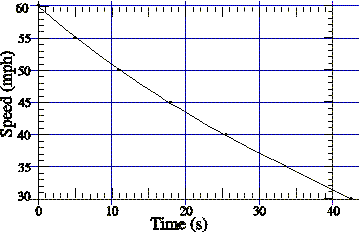# Force due to wind and rolling friction

bulldog23

## Homework Statement

The graph shows the speed as a function of time for a minivan, coasting on neutral along a straight, level road, loaded with windsurfing equipment and towing a boat.The total mass is 2450kg (weight = 5402lbs). Find the size of the force due to wind and rolling friction when the van speed is 49.27mph (1mph= 0.447m/s).## Homework Equations

delta v/delta t=a
F=ma

## The Attempt at a Solution

I converted the mph to m/s, and I drew a line tangent to the point on the graph where the speed is 49.27 mph. I just can't seem to get the numbers right for some reason. Can someone help me out?

Homework Helper
Gold Member

## Homework Statement

The graph shows the speed as a function of time for a minivan, coasting on neutral along a straight, level road, loaded with windsurfing equipment and towing a boat.The total mass is 2450kg (weight = 5402lbs). Find the size of the force due to wind and rolling friction when the van speed is 49.27mph (1mph= 0.447m/s).## Homework Equations

delta v/delta t=a
F=ma

## The Attempt at a Solution

I converted the mph to m/s, and I drew a line tangent to the point on the graph where the speed is 49.27 mph. I just can't seem to get the numbers right for some reason. Can someone help me out?
When you drew the tangent, what did you conclude about the acceleration at that point?

bulldog23
The acceleration is negative, right. So doesn't that make the force negative? The van reaches 49.27 mph at 11 sec. So what other point should I use to find the slope?

Homework Helper
Gold Member
The acceleration is negative, right. So doesn't that make the force negative? The van reaches 49.27 mph at 11 sec. So what other point should I use to find the slope?
Draw the tangent as best you can, acros the whole graph, and see what the slope is by choosing any 2 convenient points to determine the approximate acceleration ( change in v/change in t) between those points. Yes, the force will be negative.

bulldog23
Then once I get the acceleration, I just multiply by 2450 kg?

bulldog23
alright, I got it, thanks!